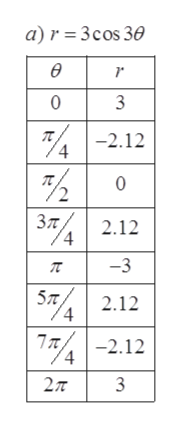# Sketch a graph of the polar equationa) r= 3cos3Θb) r=2 - 2sinΘc) r = 3cosΘ

Question
46 views

Sketch a graph of the polar equation

a) r= 3cos3Θ

b) r=2 - 2sinΘ

c) r = 3cosΘ

check_circle

Step 1

Given:

Step 2

Find the value of r by putting the value of theta:help_outlineImage Transcriptionclosea) r 3cos 30 ө r 0 3 2.12 3T 2.12 Зл, -3 ST2.12 7 2.12 27T 3 fullscreen
Step 3

Sketch the graph of polar equat...

### Want to see the full answer?

See Solution

#### Want to see this answer and more?

Solutions are written by subject experts who are available 24/7. Questions are typically answered within 1 hour.*

See Solution
*Response times may vary by subject and question.
Tagged in

### Other